All submissions of the EM system will be redirected to Online Manuscript Submission System. Authors are requested to submit articles directly to Online Manuscript Submission System of respective journal.

# Short communication

, Volume: 7( 1) DOI: 10.37532/2320-6756.2019.7(1).169

## The Electromagnetic Field of a Magnetic Dipole above a Conducting HalfSpace

*Correspondence:
Seliem Aasa Department of Mathematics, Faculty of Science, University of Kafr El-Sheikh, Egypt, E-Mail: [email protected]

Received: October 31, 2018; Accepted: November 23, 2018; Published: November 30, 2018

Citation: Adel AS Abo Seliem, Alseroury F. The Electromagnetic Field of a Magnetic Dipole Above a Conducting Half-Space. J Phys Astron. 2019;7(1):169.

### Abstract

We calculate solutions for the electromagnetic field due to a magnetic dipole and a finite loop vertically oriented and above a conducting ground by means of an approximate Green function in the frequency domain. Our solution is expressed as the sum of two partial azimuthally electric field, the two partial field are identified as radioactive and diffusion, the transient source in which the exciting current is abruptly switched off has been considered in detail.

### Keywords

Reflection; Electromagnetic field; Ward

### Introduction

The present paper is concerned priming with the asymptotic representation from the Fast Electric Maniac (FEM) above a uniform conducting ground and scattered form are bodies within the ground, the paper interpretation of their results requires the development of theoretical models as has been started by Wait JR and Kaufman AA [1,2]. The electromagnetic field due to current loop is naturally described by a magnetic Hertz vector while the elementary source is a magnetic dipole. The solution of the present boundary vale problem, where the field derived a vertically oriented distribution of magnesium above the horizontal interface of the air, and the ground is the solution. Stration considered the electromagnetic theory as section 1 , and Ward in his study concluded that a ward loop may be regarded as equivalent to the distribution of magnesium [4-6]. We consider the transient field resulting from an abrupt current and showed that the radioactive field is a superposition of poles using the intranet of the element of tangle in the ground .

The diffuse field is essentially more complex, we restrict our investigation to its structure and above the ground and lies enough for an asymptotic approximation as an application . Further, since the field is continuously ensured using a recurring loop at the same height, we restrict our calculation to the field of the height.

### Methods

The source of magnetic Hertz vectorof the distribution of magnetization M(x, t) in accordance with the vector equation of wirlas wireless telegraphy(1)

In a medium specified by its uniform permeability, permittivity and conductivity, the electric and magnetic field vectors E(x,t) and H(x,t) are given in termsby formula: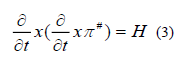In air, we takeso that equation reduced to the simpler form of the wave equation, in the ground, we takeTo satisfy the boundary conditions, the magnetic tangent component of E and H are continuous. For the horizontal loop, bothand M are parallel to unit vector k to which we take:With the origin on the surface of the ground, the boundary condition reduced to the form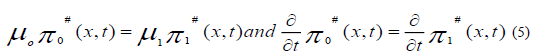On z=0 where we have taken z=x-h by applied the Fourier transform, it is convenient to eliminate time derivatives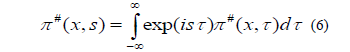Subtitled from equation (2) and (3) transforms into the scalar Helmholtz formWhere,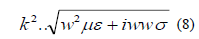The solution of the last equation subject to boundary conditions of the (5) is approximately expressed in medium terms of the Green function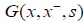corresponding to a unit source at a point x and the same boundary condition. Thus for any distribution M (x,s), we have:Where the integral is to cover the whole of space andSubstitutimg the same boundary condition transform of the field vectors are this given by the formula:We are interested in the particular case, when the sourceis a finite horizontal loop of radius ‘a’ carrying the current ‘I’ (t) at height ‘h’ above the ground taking the origin of the coordinate vertically below the loop, we haveWhere ‘c’ the integration is over the plane surface spinning the loop. The transforms of the field vectors can readily be obtained by substitution from (4) into (11) and (12) together with the application of Stokes theorem to the surface integral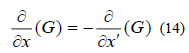Where the integration is around the loop, in the right-hand sense with respect to direction K. The Gamma function to the position can be Written in Sommerfeld form:Where,is the magnitude of the horizontal component of both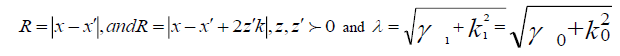Corresponding to the value of k square in the air and the ground respectively andrespectively contributions from the unit source x and its image at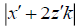have an integral representation of Euler form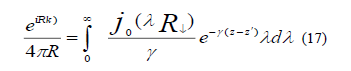We took cylindrical polar coordinatecorresponding to unit vectors and found E is independent of azimuth for having the d same direction of its interesting form. We derived the electromagnetic (emf) that would be introduced in the recurring loop, it is sufficient to investigate only the electric field due to the transmitted loop contained above the origin.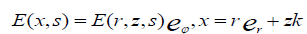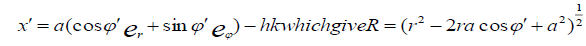And,From the addition formula for the Bessel function, the results obtained are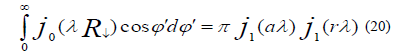When we substitute from (15) into (14), we findWhere,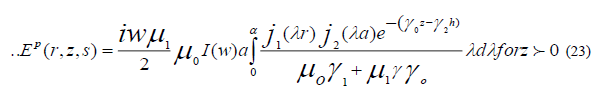The corresponding result for an elementary dipole whose mount is of limiting values offirst discussed by Wait JR , can be recovered from this formula by applying the approximations, thus, we getThe results can be obtained directly from E an substitutingthe partial field corresponding Ep (r,z,s) is purely radiated vanishing when the source is on ground, whereas that corresponding Es (r,z,s) is diffusive in character, because of its dependence on the conductive of the ground , they are given by inverse transforms , of the form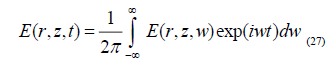In general, such field cannot be determined in exact form, however, for h=0, the radiation component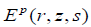is always zero owing to the coincidence of the source and image at the air-ground interface.

### Conclusions

We consider the transient field resulting from an abrupt current, we show that the relative field consists of a superposition of Huygens spectral pulse issuing at the instant of a switch - off from each element of the source and image of the ground.

### References

Welcome Message

Select your language of interest to view the total content in your interested language

###### Citations : 260

Journal of Physics & Astronomy received 260 citations as per Google Scholar report

#### Indexed In

• Open J Gate
• China National Knowledge Infrastructure (CNKI)
• Cosmos IF
• Directory of Research Journal Indexing (DRJI)
• MIAR
• Secret Search Engine Labs
• Euro Pub
• clarivate-web-of-science-logo-vector

### 9th International Conference on Physics

Edinburgh, Scotland

### 6th International Conference on Astronomy, Astrophysics and Space Science

Budapest, Hungary

Berlin, Germany

### 9th International Conference on Physics

Edinburgh, Scotland

### 6th International Conference on Astronomy, Astrophysics and Space Science

Budapest, Hungary

### World Congress on Quantum Physics

Berlin, Germany

#### For Librarians#### Open Access Journals